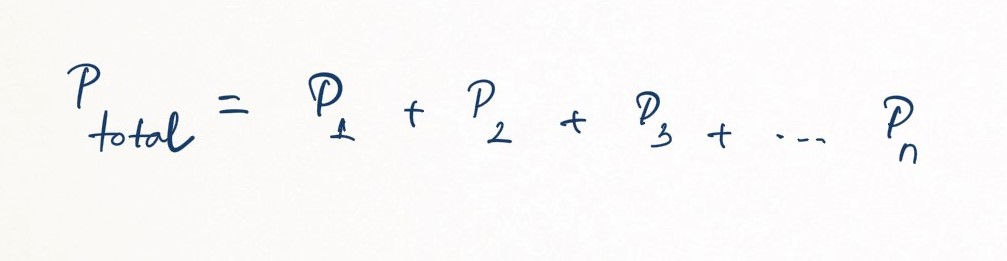Complimentary 1-hour tutoring consultation

MCAT Content / Gas Phase / Daltons Law Relating Partial Pressure To Composition

### Dalton’s Law Relating Partial Pressure to Composition

Topic: Gas Phase

Dalton’s Law of Partial Pressure states the total pressure exerted by a mixture of gases is equal to the sum of the partial pressure of each individual gas.

Because it is dependent solely on the number of particles and not the identity of the gas, the Ideal Gas Equation applies just as well to mixtures of gases as it does to pure gases. English chemist John Dalton correctly reasoned that the low density and high compressibility of gases were indicative of the fact that they consisted mostly of empty space; from this, Dalton concluded that when two or more different gases occupy the same volume, they behave entirely independently of one another.

Dalton’s Law (also called Dalton’s Law of Partial Pressures) states that the total pressure exerted by the mixture of non-reactive gases is equal to the sum of the partial pressures of individual gases. Mathematically, this can be stated as follows:where P1, P2 and Pn represent the partial pressures of each compound. It is assumed that the gases do not react with each other.

Key Points

• The total pressure of a mixture of gases can be defined as the sum of the pressures of each individual gas: .

• Boyle’s Law and the Ideal Gas Law tell us the total pressure of a mixture depends solely on the number of moles of gas, and not the kinds of molecules; Dalton’s Law allows us to calculate the total pressure in a system from each gas’ individual contribution.

Key Terms

Dalton’s Law of Partial Pressures: the total pressure exerted by the mixture of non-reactive gases is equal to the sum of the partial pressures of each individual gas.

Billing Information